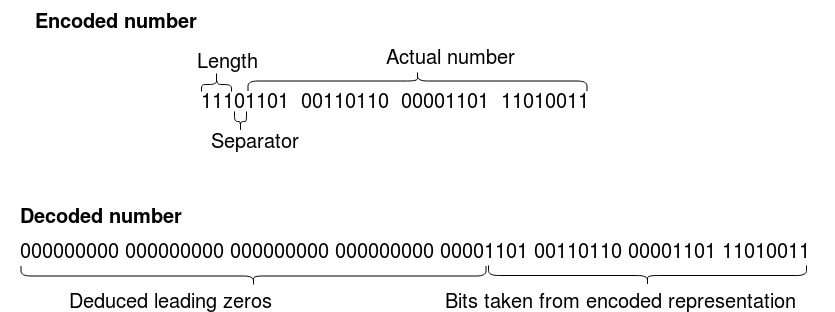# ScyllaDB/Cassandra SSTable format part 1

## Variant integers encoding

Posted by Piotr Jastrzębski on February 26, 2018

### IMPORTANT NOTE: This post describes SSTable format mc (3.0.8, 3.9). There are multiple versions of SSTable format which can differ significantly.

SSTable format uses a concept of variant integers borrowed from Protocol Buffers Base 128 Variants. Those encodings aren't exactly the same but they share the idea that an integer does not have to be represented by a fixed number of bytes. In SSTables, a variant integer can be represented by 1-9 bytes and the length is encoded in the first byte. Important characteristic of such representation is that it uses less bytes for small numbers and more bytes for large numbers than a fixed length representation. Both signed and unsigned 64-bit numbers can be translated to bytes using this encoding. Let's investigate unsigned numbers first.

# Unsigned number encoding

Table below presents the number of bytes used to represent integers from different ranges.

Range Length of byte representation
from 0 to 2^8 - 1 1
from 2^8 to 2^15 - 1 2
from 2^15 to 2^22 - 1 3
from 2^22 to 2^29 - 1 4
from 2^29 to 2^36 - 1 5
from 2^36 to 2^43 - 1 6
from 2^43 to 2^50 - 1 7
from 2^50 to 2^57 - 1 8
from 2^57 to 2^64 - 1 9

There are 3 cases we should look at:

1. Numbers that require single byte representation
2. Numbers that require 9 bytes representation
3. Numbers that require 2-8 bytes representation

## Single byte representation

Small numbers, such that are smaller than 2^8, can be represented by a single byte. The representation of such number is it's lowest byte. All the leading bytes are assumed to be 0x00. Such representation can be recognized because it has the most significant bit equal to 0. All multi byte representations have most significant bit of the first byte set to 1.

## 9 bytes representation

Big numbers, such that are bigger than 2^57 - 1, are represented using 9 bytes. First byte is 0xFF and the following 8 bytes represent the whole 64-bit number.

## 2-8 bytes representation

It takes 2 to 8 bytes to represent numbers that are bigger than 2^8-1 and smaller than 2^57. First byte starts with as many leading bits set to one as there are bytes following it in the representation. Then there is zero separating those leading ones and the actual data. All remaining bits together with the following bytes form the actual number. Those bits should be padded with leading zeros. This diagram presents an example of encoded and decoded version of a number.# Signed number encoding

Signed numbers are encoded using a ZigZag encoding and then are treated with the procedure described above. Without this additional step, it would always take 9 bytes to represent a negative number. ZigZag encoding transforms a signed number N into unsigned (N << 1) ^ (N >> 63). To recover the initial number from encoded version M (which is unsigned) we need to calculate (M >> 1) ^ -(M & 1).